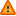FAQ/orthopolys - CBU statistics WikiYou should trust both wikis because the password could be read by the particular administrators.

Clear message
location: FAQ / orthopolys

# Fitting linear orthogonal polynomials

A linear polynomial assumes that three or more groups have an ordering e.g. A>B>C and that the distances between each successive pair of groups are equal. This may be tested by specifying contrast coefficients in an anova.

Non-integer orthogonal contrast coefficients may be obtained in R using contr.poly(p) for a polynomial of degree p. Tables of polynomial contrast coefficients are also available in Biometrika tables which also give general formulae to obtain integer contrast coefficients for comparing upto 22 group means using polynomials upto degree 6.

`contr.poly(4,contrasts=TRUE)`

Specific orthogonal contrast coefficients can also be obtained assuming unequal distances between groups but the ratio of these differences must be specified e.g. if groups B and C are twice as far from each other as groups A and B then contrast coefficients may be obtained using, for example, R.

```degree <- 2
x <- c(1,2,4)
poly(x,degree)```

If you suspect the groups are unequally spaced but do not know by how much then either fit a quadratic polynomial in addition to a linear one using a second set of contrast coefficients or use a nonparametric procedure such as Jonckheere's Trend Test.

A pair of contrasts are said to be orthogonal, or statistically independent, if the sum of their paired contrast coefficients is zero (See page 184 of Everitt (1995)). Each of the outputted contrast coefficients also sum to zero (summing across rows) and are used for fitting factors in repeated measures analysis of variance e.g. in SPSS GLM.

Orthogonal polynomials are also examples of sum to zero constraints since the sum over rows of each polynomial contrast is zero. Using sum to zero constraints is akin to centering continuous variables and similarly eliminates collinearity in ANOVAs when higher order interaction terms are present - see here.

References

Biometrika tables for Statisticians Volume 1 (1954) Edited by Pearson, ES and Hartley, HO. Cambridge University Press.

Everitt, B.S. (1995) The Cambridge Dictionary of Statistics in the Medical Sciences. Cambridge University Press.